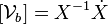# Modern Robotics Errata

Errata below are for the preprint version posted on November 29, 2016.

### Chapter 1

p4: "screw theor." should be "screw theory." (near bottom of page)

### Chapter 11

p419, the line just before equation (11.16): the expression should read$[\mathcal{V}_b] = X^{-1} \dot{X}$ (the square brackets are missing).

### Chapter 13

p518, equations (13.7) and (13.8): the rows in the matrices should be numbered to m, not n.

p550, line just after equation (13.37): the expression should read$[\mathcal{V}_d(t)] = X_d^{-1}(t) \dot{X}_d(t)$ (the square brackets are missing).